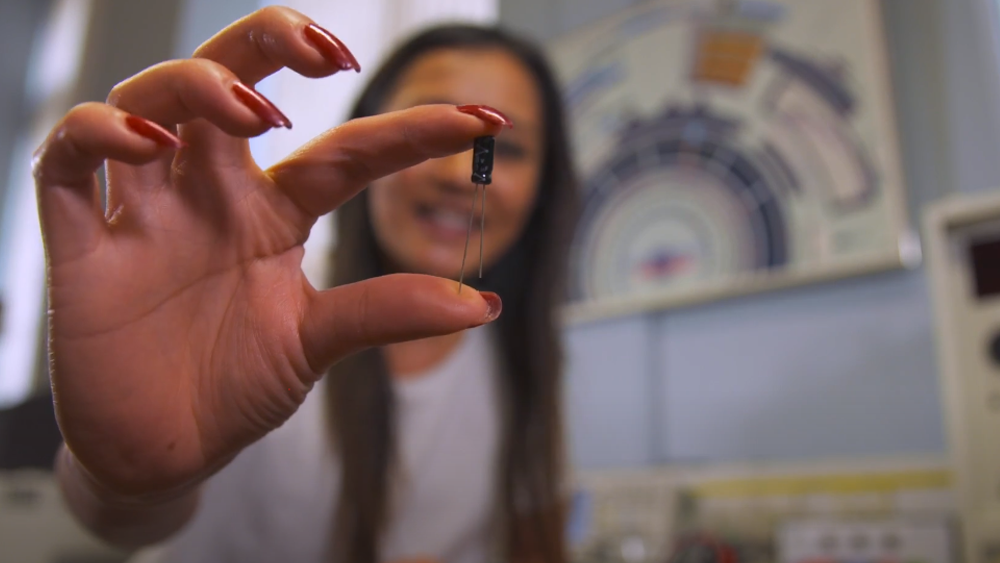# Physics A level revision resource: Introduction to capacitors

A battery stores electrical energy and releases it through chemical reactions, this means that it can be quickly charged but the discharge is slow. Unlike the battery, a capacitor is a circuit component that temporarily stores electrical energy through distributing charged particles on (generally two) plates to create a potential difference. A capacitor can take a shorter time than a battery to charge up and it can release all the energy very quickly.

## How much can we charge?

When connected to a cell or other power supply, electrons will flow from the negative end of the terminal and build up on one plate of the capacitor. The other plate will have a net positive charge as electrons are lost to the battery, resulting in a potential difference equivalent to the voltage of the cell.

A capacitor is characterised by its capacitance (C) typically given in units Farad. It is the ratio of the charge (Q) to the potential difference (V), where C = Q/V

The larger the capacitance, the more charge a capacitor can hold. Using the setup shown, we can measure the voltage as the capacitor is charging across a resistor as a function of time (t).

## How do we test the behaviour of a capacitor?A video on how to test the behaviour of a capacitor

## How do we find the time constant?

Here you can see a plot of voltage against time for charging and discharging a capacitor.

The equations of the V-t curves for the charging and discharging of a capacitor are exponential, where the voltage is proportional to the initial voltage to the power of time over capacitance. Therefore, with a bit of mathematical manipulation we can plot a log voltage - time graph of a discharging capacitor, to easily obtain the time constant (τ = RC) from the gradient (-1/RC) and the initial voltage from the y intercept.

It is really important that the capacitor used has a larger voltage rating than that of the cell and that it is connected with the right polarity (the negative plate should be connected to the negative terminal of the cell) otherwise it could potentially explode. The negative end is usually indicated by a dash on the capacitor body and is usually the shorter pin. Note however not all capacitors are polarised (usually the smaller μF ones) and can be connected in any way. Another important thing to take care of is making measurements of voltage at the set time intervals. One option would be to use an oscilloscope or to use a larger capacitor/resistor to increase the charging/discharging time.

## What do your measurements mean?

Capacitors are a common component in most electronic devices and are most importantly involved in energy storage. The development of capacitors is therefore important in order for technological advancements of batteries. Whilst current energy storage relies heavily on batteries, this may change in the future as they are slow to charge and discharge and the chemical processes involved generally causes loss of energy through heat. Although capacitors need to be made a lot larger than batteries in order to store the same amount of charge, they have significant advantages, including a much longer lifetime and non-toxic components. The effort to replace batteries with these supercapacitors and ultracapacitors is an ongoing area of research.

Previously we have said that capacitors, unlike batteries, are able to release their charge very quickly. For this reason, they have a wide application in our everyday lives and are found in nearly all household electronic devices. They are particularly useful in devices where you need a fast response, like lasers and camera flashes.

In the experiment in the video above, we have demonstrated the use of an oscilloscope to measure the time constant of a capacitor and learnt about the importance of polarity. If you have time, it would be interesting to add further components into the circuit. By adding an ammeter, we can measure the charge and hence the work done.

If we add more capacitors in series, the total capacitance is

## 1/C = 1/C1+1/C2+1/C3+...+1/CN

Where CN is the capacitance of the Nth capacitor.

Adding more capacitors in parallel gives a total capacitance of